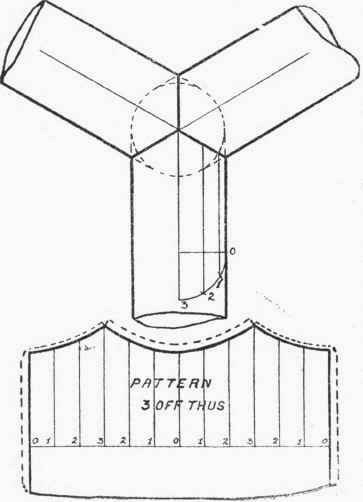When three pipes of the same diameter fit together at equal angles, as in Fig. 24, the simplest way to obtain the ele-vation, and thus the pattern, is to draw a circle (shown dotted in the figure) equal in diameter to the pipes, and obtain the elevation of the joint line, as shown. The pattern can then be set out, as in other cases. If the joint is to be paned down, or knocked up, the allowance on pattern will be a double edge for middle part and a single edge on end parts, the pattern then serving for each branch.Unequal angled Three-way Piece.

For this the elevation can be set out, as in the last case, the centre lines being drawn to the required angles. In Fig. 25 the two top pipes make equal angles with the bottom pipe, consequently one pattern will do for the two pipes, the only difference being in the arrangement of the laps, the two dotted curves on the pattern showing respectively the laps for the two pipes.Fig. 25.

If the three angles that the centre lines of the pipes make with each other are all unequal, then it will be necessary to have three distinct patterns, the setting out of these being similar to the cases already mentioned.